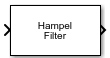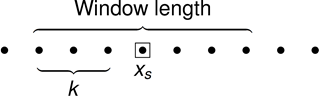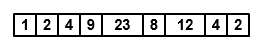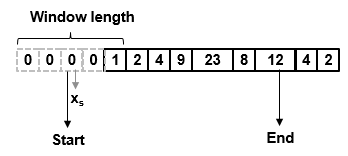# Hampel Filter

Filter outliers using Hampel identifier

• Library:
• DSP System Toolbox / Filtering / Filter Designs## Description

The Hampel Filter block detects and removes the outliers of the input signal by using the Hampel identifier. The Hampel identifier is a variation of the three-sigma rule of statistics, which is robust against outliers. For each sample of the input signal, the block computes the median of a window composed of the current sample and $\frac{Len-1}{2}$ adjacent samples on each side of the current sample. Len is the window length you specify through the Window length parameter. The block also estimates the standard deviation of each sample about its window median by using the median absolute deviation. If a sample differs from the median by more than the threshold multiplied by the standard deviation, the filter replaces the sample with the median. For more information, see Algorithms.

## Ports

### Input

expand all

The block accepts multichannel inputs, that is, m-by-n size inputs, where m ≥ 1, and n ≥ 1. m is the number of samples in each frame (channel), and n is the number of channels. The block also accepts variable-size inputs. That is, you can change the size of each input channel during simulation. However, the number of channels cannot change.

This port is unnamed until you select the Specify threshold from input port parameter.

Data Types: `single` | `double`

Threshold for outlier detection, specified as a real scalar greater than or equal to 0. For information on how this parameter is used to detect the outlier, see Algorithms.

#### Dependencies

This port appears when you select the Specify threshold from input port parameter.

Data Types: `single` | `double`

### Output

expand all

The size and data type of this output matches the size and data type of the input.

This port is unnamed until you select the Output outlier status check box.

Data Types: `single` | `double`

A value of `1` in this output indicates that the corresponding element in the input is an outlier. This output has the same size as the input.

#### Dependencies

To enable this port, select the Output outlier status check box.

Data Types: `Boolean`

## Parameters

expand all

If a parameter is listed as tunable, then you can change its value during simulation.

Length of the sliding window, specified as a positive odd scalar integer. The window of finite length slides over the data, and the block computes the median and median absolute deviation of the data in the window.

When you select this check box, the threshold is input through the T port. When you clear this check box, the threshold is specified on the block dialog through the Threshold for outlier detection (standard deviations) parameter.

Threshold for outlier detection, specified as a real scalar greater than or equal to 0. For information on how this parameter is used to detect the outlier, see Algorithms.

Tunable: Yes

#### Dependencies

This parameter appears when you clear the Specify threshold from input port check box.

Select this parameter to output a matrix of `boolean` values that has the same size as the input. Each element in this matrix indicates whether the corresponding element in the input is an outlier. A value of `1` indicates an outlier.

• `Interpreted execution`

Simulate model using the MATLAB®  interpreter. This option shortens startup time and provides faster simulation speed than `Code generation`.

• `Code generation`

Simulate model using generated C code. The first time you run a simulation, Simulink® generates C code for the block. The C code is reused for subsequent simulations, as long as the model does not change. This option requires additional startup time and has slower simulation speed than ```Interpreted execution```.

## Block Characteristics

 Data Types `double` | `single` Direct Feedthrough `no` Multidimensional Signals `no` Variable-Size Signals `yes` Zero-Crossing Detection `no`

expand all

## AlgorithmsFor a given sample of data, xs, the algorithm:

• Centers the window of odd length at the current sample.

• Computes the local median, mi, and standard deviation, σi, over the current window of data.

• Compares the current sample with nσ × σi, where nσ is the threshold value. If $|{x}_{s}-{m}_{i}|>{n}_{\sigma }×{\sigma }_{i}$, the filter identifies the current sample, xs, as an outlier and replaces it with the median value, mi.

Consider a frame of data that is passed into the Hampel filter.In this example, the Hampel filter slides a window of length 5 (Len) over the data. The filter has a threshold value of 2 (nσ). To have a complete window at the beginning of the frame, the filter algorithm prepends the frame with Len – 1 zeros. To compute the first sample of the output, the window centers on the ${\left[\frac{Len-1}{2}+1\right]}^{\text{th}}$ sample in the appended frame, the third zero in this case. The filter computes the median, median absolute deviation, and the standard deviation over the data in the local window.• Current sample: xs = 0.

• Window of data: win = [0 0 0 0 1].

• Local median: mi = median([0 0 0 0 1]) = 0.

• Median absolute deviation: $ma{d}_{i}=\mathrm{median}\left(|{x}_{i-k}-{m}_{i}|,\dots ,|{x}_{i+k}-{m}_{i}|\right)$. For this window of data, $mad=\mathrm{median}\left(|0-0|,\dots ,|1-0|\right)=0$.

• Standard deviation: σi = κ × madi = 0, where $\kappa =\frac{1}{\sqrt{2}{\mathrm{erfc}}^{-1}\left(1/2\right)}\approx 1.4826$.

• The current sample, xs = 0, does not obey the relation for outlier detection.

`$\left[|{x}_{s}-{m}_{i}|=0\right]>\left[\left({n}_{\sigma }×{\sigma }_{i}\right)=0\right]$`

Therefore, the Hampel filter outputs the current input sample, xs = 0.

Repeat this procedure for every succeeding sample until the algorithm centers the window on the ${\left[End-\frac{Len-1}{2}\right]}^{\text{th}}$ sample, marked as `End`. Because the window centered on the last $\frac{Len-1}{2}$ samples cannot be full, these samples are processed with the next frame of input data.

Here is the first output frame the Hampel filter generates:The seventh sample of the appended input frame, 23, is an outlier. The Hampel filter replaces this sample with the median over the local window [4 9 23 8 12].

 Bodenham, Dean. “Adaptive Filtering and Change Detection for Streaming Data.” Ph.D. Thesis. Imperial College, London, 2012.

 Liu, Hancong, Sirish Shah, and Wei Jiang. “On-line outlier detection and data cleaning.” Computers and Chemical Engineering. Vol. 28, March 2004, pp. 1635–1647.

Watch now